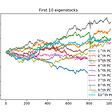# Coding a Neural Network from Scratch

`import numpy as numpy# Source: https://victorzhou.com/blog/intro-to-neural-networks/?fbclid=IwAR1cCqR8kcJm9Ui8uo5FLWzJA2lVVqWzMVv7twy1NQCogydDbUXDwvZ1jQg###### Neuronsdef sigmoid(x):# Our activation function is: f(x) = 1 / (1 + e^(-x))return 1 / (1 + numpy.exp(-x))class Neuron:def __init__(self, weights, bias):self.weights = weightsself.bias = biasdef feedforward(self, inputs):# Weight inputs, add bias, then use the activation functiontotal = numpy.dot(self.weights, inputs) + self.biasreturn sigmoid(total)# w1 = 0, 22 = 1weights = numpy.array([0,1])# b = 4bias = 4n = Neuron(weights,bias)# x1 = 2, x2 = 3x = numpy.array([2,3])print('Neuron: n.feedforward(x)')# 0.9990889488055994 ~= 1print(n.feedforward(x))print()###### Neural Networkclass MyNeuralNetwork:'''A neural network with:- 2 inputs- a hidden layer with 2 neurons (h1, h2)- an output layer with 1 neuron (o1)Each neuron has the same weights and bias:- w = [0, 1]- b = 0'''def __init__(self):# weights = numpy.array([0,1])# bias = 0# self.h1 = Neuron(weights, bias)# self.h2 = Neuron(weights, bias)# self.o1 = Neuron(weights, bias)# Weightsself.w1 = numpy.random.normal()self.w2 = numpy.random.normal()self.w3 = numpy.random.normal()self.w4 = numpy.random.normal()self.w5 = numpy.random.normal()self.w6 = numpy.random.normal()# Biasesself.b1 = numpy.random.normal()self.b2 = numpy.random.normal()self.b3 = numpy.random.normal()def feedforward(self, x):# x is a numpy array with 2 elements# h1_output = self.h1.feedforward(x)h1 = sigmoid(self.w1 * x + self.w2 * x + self.b1)# h2_output = self.h2.feedforward(x)h2 = sigmoid(self.w3 * x + self.w4 * x + self.b2)# The inputs for o1 are the outputs from h1 and h2# o1_output = self.o1.feedforward(numpy.array([h1_output, h2_output]))o1_output = sigmoid(self.w5 * h1 + self.w6 * h2 + self.b3)return o1_outputdef train(self, data, all_y_trues):# - data is a (n x 2) numpy array, n = # of samples in the dataset# - all_y_trues is a numpy array with n elements# Elements in all_y_trues correspond to those in datalearn_rate = 0.1# Number of times to loop through the entire datasetepochs = 1000for epoch in range(epochs):for x, y_true in zip(data, all_y_trues):# --- Do a feedforward (we'll need these values later)sum_h1 = self.w1 * x + self.w2 * x + self.b1h1 = sigmoid(sum_h1)sum_h2 = self.w3 * x + self.w4 * x + self.b2h2 = sigmoid(sum_h2)sum_o1 = self.w5 * h1 + self.w6 * h2 + self.b3o1 = sigmoid(sum_o1)y_pred = o1# --- Calculating partial derivatives# Naming: d_L_d_w1 represents "partial L / partial w1"d_L_d_ypred = -2 * (y_true - y_pred)# Neuron o1d_ypred_d_w5 = h1 * deriv_sigmoid(sum_o1)d_ypred_d_w6 = h2 * deriv_sigmoid(sum_o1)d_ypred_d_b3 = deriv_sigmoid(sum_o1)d_ypred_d_h1 = self.w5 * deriv_sigmoid(sum_o1)d_ypred_d_h2 = self.w6 * deriv_sigmoid(sum_o1)# Neuron h1d_h1_d_w1 = x * deriv_sigmoid(sum_h1)d_h1_d_w2 = x * deriv_sigmoid(sum_h1)d_h1_d_b1 = deriv_sigmoid(sum_h1)# Neuron h2d_h2_d_w3 = x * deriv_sigmoid(sum_h2)d_h2_d_w4 = x * deriv_sigmoid(sum_h2)d_h2_d_b2 = deriv_sigmoid(sum_h2)# --- Update weights and biases# Neuron h1self.w1 -= learn_rate * d_L_d_ypred * d_ypred_d_h1 * d_h1_d_w1self.w2 -= learn_rate * d_L_d_ypred * d_ypred_d_h1 * d_h1_d_w2self.b1 -= learn_rate * d_L_d_ypred * d_ypred_d_h1 * d_h1_d_b1# Neuron h2self.w3 -= learn_rate * d_L_d_ypred * d_ypred_d_h2 * d_h2_d_w3self.w4 -= learn_rate * d_L_d_ypred * d_ypred_d_h2 * d_h2_d_w4self.b2 -= learn_rate * d_L_d_ypred * d_ypred_d_h2 * d_h2_d_b2# Neuron o1self.w5 -= learn_rate * d_L_d_ypred * d_ypred_d_w5self.w6 -= learn_rate * d_L_d_ypred * d_ypred_d_w6self.b3 -= learn_rate * d_L_d_ypred * d_ypred_d_b3# --- Calculate total loss at the end of each epochif epoch % 10 == 0:y_preds = numpy.apply_along_axis(self.feedforward, 1 , data)loss = mse_loss(all_y_trues, y_preds)print("Epoch %d loss: %.3f" % (epoch, loss))network = MyNeuralNetwork()x = numpy.array([2,3])print('Network: network.feedforward(x)')# 0.7216325609518421print(network.feedforward(x))print()###### Training a neural network, part 1def mse_loss(y_true, y_predicted):# y_true and y_predicted are numpy arrays of the same lengthreturn ((y_true - y_predicted) ** 2).mean()y_true = numpy.array([1,0,0,1])y_predicted = numpy.array([0,0,0,0])print('Mean Square Error Loss: mse_loss(y_true, y_predicted)')# 0.5print(mse_loss(y_true, y_predicted))print()##### A complete neural networkdef deriv_sigmoid(x):# Derivative of sigmoid: f'(x) = f(x) * (1 - f(x))fx = sigmoid(x)return fx * (1 - fx)# Define datasetdata = numpy.array([[-2, -1],  # Alice[25, 6],   # Bob[17, 4],   # Charlie[-15, -6], # Diana])all_y_trues = numpy.array([1, # Alice0, # Bob0, # Charlie1, # Diana])# Train our neural network!network = MyNeuralNetwork()network.train(data, all_y_trues)`

--

--

--

## More from Lawson Hung

Software Engineer

Love podcasts or audiobooks? Learn on the go with our new app.

## Applying PCA to Stocks## Kaggle Competition : Google Analytics Customer Revenue Prediction## Understanding ML In Production: On Apache Beam## How to design a high-performance neural network on a GPU## How to Tackle Multicollinearity## Build Our First Convolutional Neural Network## Machine Learning and Civil Liberties## Lawson Hung

Software Engineer

## Why We Should Learn a Programming Language Like Python?## HOW TO GENERATE A NEGATIVE IMAGE IN PYTHON USING OPENCV — INTERESTING PROJECT## How To join my Deep Learning and Computer Vision Course for Free## Deep Dive: Classification Neural Networks